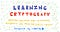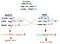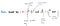This is Episode 2 in the Learning Cryptography series.

# Introduction

Diffie-Hellman Key Exchange is used for various public key/private key encryption schemes. Security assumptions about the key exchange protocol are guaranteed through the difficulty of breaking the discrete log problem. We will talk about how we can generate discrete logarithmic problems through generator points of polynomials of extension fields (covered in the previous article).

If you haven’t read the previous article about finite fields, I’d highly recommend you to do so before reading this article!

# Background

Loopring’s “Learning Cryptography” series hopes to educate the community about this fascinating field. This series will begin from the basics, and work its way up to the advanced tools that make our scalable v3 DEX protocol — which utilizes zero-knowledge proofs — possible.

# Diffie-Hellman Key Exchange

Suppose Bob wanted to communicate with Alice in a secure way. To keep things simple, they could have a shared secret between them which could both agree on and encrypt all their messages with that secret. However, there are two problems with this scheme:

1. How do they communicate the secret in a secret way?
2. How is key abuse prevented? eg. Alice and Bob may share the keys with 3rd parties.

What we’re describing here is a symmetric key encryption scheme. The trade-offs we described above don’t really cut it for adversarial environments such as crypto. So what’s the solution? Asymmetric key encryption schemes.

Diffie-Hellman is an asymmetric key exchange protocol where each party has its own public key and private key.

Suppose:

• Alice has her private key `a` and public key `A` and Bob has his private key `b` and public key `B`;
• We have two variables which are known as public parameters: `p` (some large prime number) and `𝛂` (some integer);
• private keys `a` and `b` are randomly selected numbers from a finite field having `p-1` elements;Diffie-Hellman Key Exchange

To send a message to Bob, Alice would:

1. Compute her public key `A` through the equation `A=𝛂^a mod p`. `𝛂` is our public variable integer, the exponent is `a` (Alice’s private key);
2. Alice then sends her public key `A` to Bob. You might be thinking that couldn’t Bob rework the equation to obtain Alice’s private key, rest assured this isn’t possible and we’ll explain why later on;
3. Bob computes his public key `B` through the equation `B=𝛂^b mod p`. `𝛂` is our public variable integer, the exponent is `b` (Bob’s private key) ;
4. Bob sends his public key`B` to Alice as well;
5. Now, Alice has a key `K` defined as `B^a` and Bob also has the same key `K` defined as `A^b`;
6. Alice and Bob can securely chat with each other by encrypting all their messages `x` (secret message) with `K` generating ciphertexts `c` (garbled mess).

Point 4 probably threw you off. How can `B^a` and `A^b` be the same as you ask? Let’s break down the math:

• `A^b` can also be rewritten as `(α^a)^b`Edit: “A” should be labeled “Alice’s public key”, not private key.
• `B^a` is actually the same as`(𝛂^b)^a` as shown in step 3
• `(𝛂^a)^b` is the same as `(α^b)^a`

Since each party can independently generate `(𝛂^b)^a` or `(𝛂^a)^b` without knowing the other party’s private key, they can independently decipher the cipher-text `c` (garbled mess) to find out `x` (secret message).

There’s still one unanswered question: if Alice gives Bob `A` which is defined as `A=𝛂^a mod p`, couldn’t Bob find out what `a` is since he already knows`A`, `p` and `𝛂`? The answer is: not until quantum computers come out.

# Discrete Log Problem

To understand the discrete logarithm problem, let’s try to solve a simple equation:`19683 = 3^n`.

If you opened up your calculator you can solve this by simply entering `log(19683)/log(3)` which gives us 9 — not too hard at all. However, if we said that this equation was the following instead, you’d have a much harder time solving it:

`g^y = x mod p`, where `g`, `y`, `p`, and `x` are certain prime numbers and `y` is unknown.

Cryptography as we know it today relies on the difficulty of solving this equation (which is near impossible). Achieving computation difficulty for the discrete logarithm problem relies on choosing large and difficult prime numbers. I’ll go deeper into this voodoo magic down the line.

# Cyclic Groups

Groups in cryptography refer to a set of elements that are all strongly related to each other. An example would be` Z*5={1, 2, 3, 4}`. However, what makes them special is if you perform the following operations you’ll always end up with elements inside the group:

`2^1=2   mod 5 = 22^2=4   mod 5 = 42^3=8   mod 5 = 32^4=16  mod 5 = 12^5=32  mod 5 = 22^6=64  mod 5 = 42^7=128 mod 5 = 3...`

In this case, we refer to `2` as a generator point inside the cyclic group `Z*5`. `3` is another generator point for this cyclic group. In order for a group to cyclic, it must have at least one generator point. All finite fields with multiplicative groups are cyclic.

Going back to our equation `g^y = x mod p`, the problem is only difficult if:

• `g` is a base generator in some cyclic group `p`
• `p` is a prime number that has the true security, of some number n, `n / 2` bits (compared to RSA which needs thousands of bits for the same level of security)

# Closing

Hopefully, by now you understand what Diffie-Hellman key exchange is, how it relates to the discrete logarithm problem and why we need cyclic groups to guarantee the security of it all. Stay tuned for the next article in the series!

# About Loopring

Loopring is a decentralized exchange protocol utilizing zkSNARKs to bring highly scalable, non-custodial trading to the masses. You can sign up for their bi-weekly update, and learn more at:

⭑ Twitter: twitter.com/loopringorg
⭑ Reddit: reddit.com/r/loopringorg
⭑ Telegram: t.me/loopring_en & t.me/loopringfans (Chinese)
⭑ Discord: discord.gg/KkYccYp
⭑ GitHub: https://github.com/Loopring
⭑ Kakao: open.kakao.com/o/gJbSZdF (Korean)

Written by

## Loopring Protocol

#### Loopring Official Blog

Welcome to a place where words matter. On Medium, smart voices and original ideas take center stage - with no ads in sight. Watch
Follow all the topics you care about, and we’ll deliver the best stories for you to your homepage and inbox. Explore
Get unlimited access to the best stories on Medium — and support writers while you’re at it. Just \$5/month. Upgrade